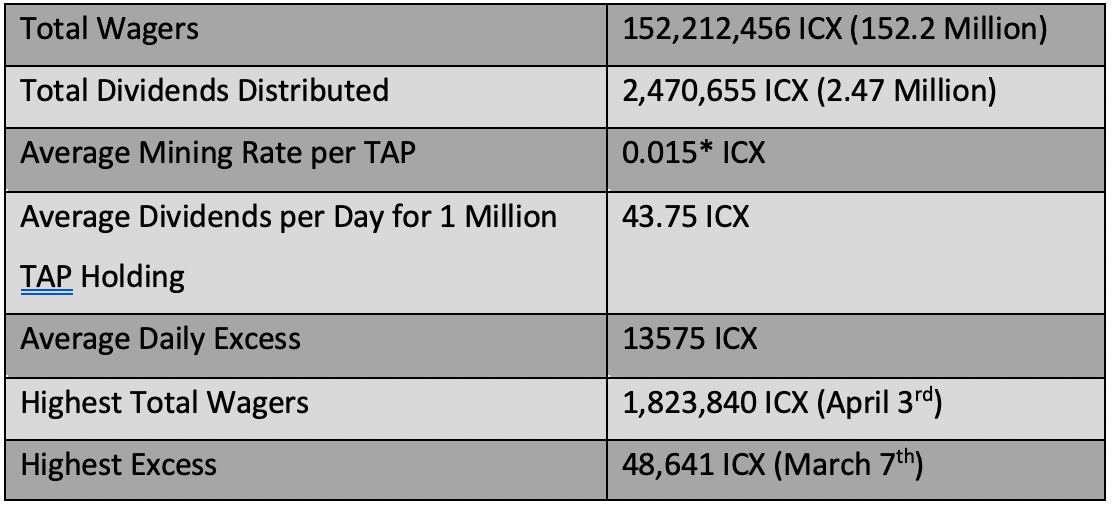# ICONbet Statistics for the period January 1st,2020 — June 30th, 2020

The below statistics have been compiled using the publicly available data and the data shared by the ICONbet community member.*Average mining rate is calculated based on just the excess, if the developer fee is to be considered the average mining rate would be up to 20% more.

If you had 1 Million TAP by Dec 31st,2019, you would have made 7962 ICX in the following 6 Months.

Following would be your ROI based on your cost of acquiring TAP.

If cost of 1 Million TAP = 13000 ICX.
ROI for 6 Months would be =(7962/13000)*100 =61.24%

If cost of 1 Million TAP = 14000 ICX.
ROI for 6 Months would be =(7962/14000)*100 =56.87%

If cost of 1 Million TAP = 15000 ICX.
ROI for 6 Months would be =(7962/15000)*100 =53.08%

If cost of 1 Million TAP = 16000 ICX.
ROI for 6 Months would be=(7962/16000)*100 =49.76%

Note: If you like what we are doing, please vote for ICONbet Community P-Rep Node. Every vote and every voter counts.

https://iconbet.io/